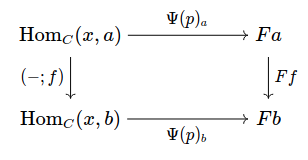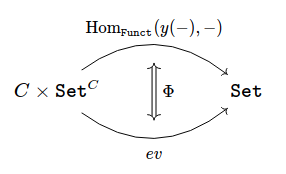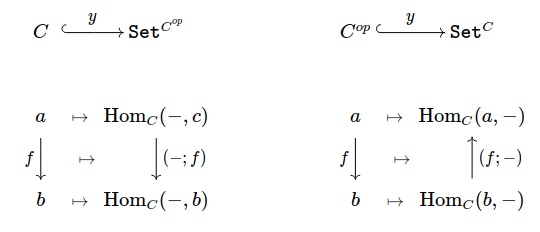# Yoneda Lemma

## Yoneda Lemma

Note: This post is mostly a retelling of [Riehl 2017:2.3] and [Milewski 2015], with quite a few missing details filled in and a number of extra examples.

As discussed by [Milewski 2015],

many constructions in category theory generalize results from other, more specific, areas of mathematics. The Yoneda Lemma stands out in this respect as a sweeping statement about categories in general, with little or no precedent in other areas of mathematics, but with far-reaching implications.

For this reason, the Yoneda Lemma is called by some the "first" theorem in category theory.

### Prerequisites

• co/contravariant [[hom-functors]] act on Hom-sets by post/precomposition
• [[representable-functors]] are naturally isormorphic to $\Hom_C(x,-)$ or $\Hom_C(-,y)$

### Introduction

Quoted from Milewski

We have seen previously that some $\Set$-valued functors are [[representable]], being naturally isomorphic to a co/contravariant [[hom-functor]]. The Yoneda Lemma reveals that all $\Set$-valued functors can be obtained from hom-functors through natural transformations, and explicitly enumerates all such transformations.

In fact, a natural transformation between a hom-functor and any functor $F : C \rightarrow \Set$ is completely determined by specifying the value of a single component at just one point! The rest of the natural transformation follows from naturality conditions, which evidently are quite restrictive!

Moreover, the Yoneda Lemma helps to clarify the relationship between representable functors and [[universal-properties]] by answering the following questions (Riehl):

• If two objects represent the same functor, are they isomorphic?
• What data is involved in the construction of a natural isomorphism between a representable functor $F : C \rightarrow \Set$ and the functor $\Hom_C(c,-) : C \rightarrow \Set$ represented by an object $c$?
• How do the universal properties expressed by representable functors relate to initial and [[terminal-objects]] ?

The Yoneda Lemma is often stated in the language of [[presheaves]] . There is also a version Yoneda Lemma for [[enriched-categories]].

### Informal Characterizations of Yoneda

We have previously seen that

Roughly, the Yoneda lemma says that an object in a category is no more and no less than its web of relationships with other objects, formalizing the idea that categories allow for [[point-free]] reasoning.

From [[milewski:ct4p-yoneda]] ,

One way of understanding the Yoneda lemma is to realize that natural transformations between Set-valued functors are just families of functions, and functions are in general lossy -- a function may collapse information and it may cover only parts of its codomain.

The only functions that are not lossy are the ones that are invertible — the isomorphisms. It follows then that the best structure-preserving Set-valued functors are the representable ones. They are either the hom-functors or the functors that are naturally isomorphic to hom-functors.

Any other functor F is obtained from a hom-functor through a lossy transformation. Such a transformation may not only lose information, but it may also cover only a small part of the image of the functor F in Set.

### Formal Statement

Let $C$ be a locally small category. According to [Riehl 2017], natural transformations $\eta : \Hom_C(x,-) \Rightarrow F$ whose domain is a represented functor are completely determined by the choice of a single element, which lives in the set $Fx \in \Set$ defined by evaluating the codomain functor $F : C \rightarrow \Set$ at the representing object $x \in C$, and moreover any choice is permitted.

(Yoneda Lemma) Consider a locally small category $C$. For any object $x \in C$ and any (covariant) functor $F : C \rightarrow \Set$, there is a bijection $\Phi$ of sets

$[C,\Set]( \Hom_C(x,-), F) \cong Fx$

that associates each natural transformation $\eta : \Hom_C(x,-) \Rightarrow F$ with the element $\Phi(\eta) \equiv \eta_x(\id_x) \in Fx$. Moreover, this correspondence is natural in both $x$ and $F$.

We prove the bijection $\Phi$ by manually constructing an inverse $\Psi$ and showing that the two maps are mutually inverse. For naturality, see [Riehl 2017], Sec 2.2.

• The inverse map $\Psi : Fx \rightarrow [C,\Set](\Hom_C(x,-),F)$ must send each $p \in Fx$ to a natural transformation $\Psi(p) : \Hom_C(x,-) \Rightarrow F$, with component morphisms satisfying the naturality squares depicted below, for all $f : a \rightarrow b$ in $C$.• To define $\Psi(p) : \Hom_C(x,-) \Rightarrow F$ for a particular $p \in Fx$, consider the image of the morphism $\id_x \in \Hom_C(x,x)$ wrt each path in the naturality square above. Our choice is constrained by the following naturality condition: $\Psi(p)_b f = (Ff)( {\color{green} \underline{\Psi(p)_x \id_x}} ) \qquad \forall\, f : x \rightarrow b, \forall\, p \in F x$

• Our inverse map must obey $\Phi(\Psi(p)) = p$ for all $p \in Fx$. Applying our explicit formula for $\Phi$ results in following constraint on $\Psi$, $\Phi(\Psi(p)) = {\color{green} \underline{\Psi(p)_x \id_x} } = p \quad \forall\, p \in Fx$

• These two constraints uniquely determine the inverse map, $\Psi(p)_b f = (F f) p \qquad \forall p \in Fx, b \in C$

• This choice of $\Psi(p)$ guarantees that the naturality squares for any $f : x \rightarrow b$ commute, but we must verify naturality for generic $f : a \rightarrow b$ with $a,b \in C$. So, consider the image of any $g : x \rightarrow a$ along both paths. \begin{aligned} \text{\color{gray}(top-right)} && (Ff)(\Psi(p)_a g) &= (Ff)(Fg)(p) \\ &&&= F(fg) p = \Psi(p)_b (g ; f) && \text{\color{gray}(left-bottom)} \end{aligned}

Hence $\Psi(p)$ is a valid natural transformation. To show $\Psi$ and $\Phi$ are mutually inverse,

• By construction $\Phi(\Psi(p)) = \Psi(p)_x (\id_x) = F (\id_x) p = \id_{Fx}(p) = p$.
• To show $\Psi(\Phi(\eta)) = \eta$ for all natural transformations $\eta : \Hom_C(x,-) \Rightarrow F$, we check componentwise equality. For all $f : a \rightarrow b$, \begin{aligned} \Psi(\Phi(\eta))_a f &= \Psi(\eta_x (\id_x))_a f & \text{(expand \Phi)} \\ &= (F f)(\eta_x (\id_x)) & \text{(expand \Psi)} \\ &= \eta_a (f) & \text{(naturality of \eta)} \end{aligned} The last step follows from the naturality square for $\eta$ and $f : a \rightarrow b$ .

### CoYoneda Lemma

There is an analogous coYoneda Lemma for contravariant functors.

(coYoneda Lemma) Consider a locally small category $C$. For any object $x \in C$ and any contravariant functor $F : C^{op} \rightarrow \Set$, there is a bijection $\Phi$ of sets

$[C^{op},\Set]( \Hom_C(-,x), F) \cong Fx$

Apply the Yoneda Lemma to $C^{op}$. Formulas for the forward $\Phi(\eta) = \eta_x(id_x)$ and reverse $\Psi(p)_b h = (Fh) p$ bijections are the same as before.

## Yoneda Embedding

Let $C$ be a locally small category. An important special case of the Yoneda lemma is when $F = \Hom_C(-,y)$ is another [[hom-functor]], leading us to the Yoneda Embeddings. The following definition is a special case of the bijection $\Psi$ used in the Yoneda proof.

The (covariant) Yoneda Embedding is the covariant functor

\begin{aligned} Y : C &\rightarrow [C^{op}, \Set] \\ x &\mapsto \Hom_C(-,x) & \text{(objects)} \\ (f : x \rightarrow y) &\mapsto (- \fcmp f) & \text{(morphisms)} \end{aligned}

sending each object to its contravariant Hom-functor, and each morphism $(f : x \rightarrow y)$ to the natural transformation $(-\fcmp f)$ defined by the $f$-postcomposition.

See [[hom-functor]] for details about the natural transformation $(- \fcmp f)$. Before proceeding, we should verify that the map $Y$ is indeed a covariant functor.

The morphisms $f : x \rightarrow y$ and $g : y \rightarrow z$ in $C$ are sent by the Yoneda embedding to the natural transformations

\begin{aligned} Yf &= (- \fcmp f) : \Hom_C(-,x) \Rightarrow \Hom_C(-,y) \\ Yg &= (- \fcmp g) : \Hom_C(-,y) \Rightarrow \Hom_C(-,z) \\ \end{aligned}

We must show $Y(f \fcmp g) = (Yf \fcmp Yg)$, where the composition $(f \fcmp g) : x \rightarrow z$ has image

$Y(f \fcmp g) = (- \fcmp (f \fcmp g)) : \Hom_C(-,x) \Rightarrow \Hom_C(-,z)$

whose components $(- \fcmp (f \fcmp g))_c : \Hom_C(c,x) \rightarrow \Hom_C(c,z)$ act on morphisms $h : c \rightarrow x$ as

\begin{aligned} \left[ Y (f \fcmp g) \right]_c h &= (- \fcmp (f \fcmp g))_c h \\ &= (h \fcmp (f \fcmp g)) \\ &= ((h \fcmp f)) \fcmp g) \\ &= (- \fcmp g)_x (h \fcmp f) \\ &= (- \fcmp g)_x (- \fcmp f)_c h = [ Yf \fcmp Yg ]_c h \end{aligned}

where the middle step relies on associativity of function composition.

The most useful fact about the Yoneda functor is that it is [[fully-faithful]], meaning that we always have a bijection between hom-sets in the domain and image of $Y$.

For a locally small category $C$, the Yoneda Embedding $Y : C \rightarrow [C^{op},\Set]$ is a [[fully-faithful]] functor, embedding $C$ as a [[full-subcategory]] of $[C^{op}, \Set]$.

We must show that the maps $\Hom_C(x,y) \stackrel{Y}{\rightarrow} [C^{op},\Set](\Hom_C(-x,), \Hom_C(-,y))$ are surjective (full) and injective (faithful) for all $x,y \in C$. Choosing $F := \Hom_C(-,y)$ in the coYoneda Lemma gives a bijection $\Psi$ between these same sets.

We claim $Y = \Psi$. The inverse bijection $\Psi$ maps each morphism $f : x \rightarrow y$ to a natural transformation whose component morphisms $\Psi(f)_c : \Hom_C(c,x) \rightarrow \Hom_C(c,y)$ act on morphisms $h : c \rightarrow x$ in $C$ by the formula

$\Psi(f)_c h = \left[ \Hom_C(-,y) h \right] f = (- \fcmp h) f = (f \fcmp h) = (f \fcmp -)_c h$

Thus $\Psi f = (f \fcmp -) = Yf$ coincides with the Yoneda embedding on $\Hom_C(x,y)$. Since $\Psi$ is a bijection, the maps $Y|_{x,y}$ are injective and surjective for all $x,y \in C$.

By now, it should be clear that we did not pull the Yoneda embedding out of thin air -- instead, $Y$ is the result of applying the Yoneda Lemma to the functors $\Hom_C(-,x)$ and $\Hom_C(-,y)$ for all pairs $x,y \in C$, and assembling the resulting bijections into a functor.

In a locally small category, $\Hom_C(x,-) \cong \Hom_C(y,-) \iff x \cong y$.

Every [[fully-faithful]] functor reflects isomorphisms.

### Contravariant Yoneda Embedding

Naturally, there is a contravariant Yoneda embedding found by applying the above to $C^{op}$

[[todo]] : according to a comment on Milewski's blog, the coYoneda embedding functor is also known as the [[free-functor]].

The contravariant Yoneda Embedding is the contravariant functor

\begin{aligned} Y : C^{op} &\rightarrow [C, \Set] \\ x &\mapsto \Hom_C(x,-) & \text{(objects)} \\ (f : x \rightarrow y) &\mapsto (f \fcmp -) & \text{(morphisms)} \end{aligned}

sending each object to its covariant [[hom-functor]] and each morphism $(f : x \rightarrow y)$ to the natural transformation $(f \fcmp -)$ defined by $f$-precomposition. The functor $Y$ is [[fully-faithful]] and embeds $C^{op}$ as a [[full-subcategory]] of $[C, \Set]$. Consequently,

$\Hom_C(x,-) \cong \Hom_C(y,-) \iff x \cong y$

### Yoneda and Universal Elements

See [[universal-element]] for the connection between Yoneda and [[universal-properties]].

### Yoneda Embedding / Evaluation

this section is incomplete ! [[TODO]]

The pair $(x,F)$ in the statement of the Yoneda Lemma defines an object in the product category $C \times \Set^C$, where $\Set^C$ denotes the category of functors $C \rightarrow \Set$ and natural transformations between them.

We can think of the bijection maps $\Phi_{(x,F)}$ used in the Yoneda Lemma as components of a natural isomorphism $\Phi$ between two functors we will now introduce.

• The codomain of $\Phi$ is the covariant bifunctor $\ev : C \times \Set^C \rightarrow \Set$ defined by the evaluation map $(x,F) \mapsto F x$.
• The domain is the covariant bifunctor $G : (x,F) \mapsto \Hom_{Funct}(\Hom_C(x,-),F)$

For details of how the domain functor is constructed, see [Riehl 2017]. The Yoneda Lemma guarantees that this bifunctor is valued in the category of sets. Now, taking the Yonda Lemma together for each $(x,F)$ pair asserts that evaluating the representing object's identity morphism defines a natural isomorphismHence every locally small category $C$ is isomorphic to the full subcategory of $\Set^{C^{op}}$ spanned by the contravariant represented functors and likewise, $C^{op}$ is isomorphic to the full subcategory of $\Set^C$ spanned by the covariant represented functors, via the canonically-defined covariant and contravariant Yoneda embeddings.

(Yoneda Embedding) The functors below are full and faithful embeddings.That is, natural transformations between represented functors correspond to morphisms between representing objects!

## Applications of Yoneda

everything you need to know about the Yoneda Lemma is encoded by its relationship with other topics! :)

### Mathematics

Let $(P, \leq)$ be a [[preorder]] category, where each hom-set contains at most one morphism, and where each morphism $f : x \rightarrow y$ can be thought of as [[evidence]] that the statement $(x \leq y)$ is true. The Yoneda embedding reads

$[P, \Set]\bigg( (x \leq -), (y \leq -) \bigg) \cong (y \leq x)$

Using [[coq]] syntax, the left-hand side is the set of polymorphic functions which convert evidence that $(x \leq z)$ into evidence that $(y \leq z)$, that is,

η : forall z, (x <= z) -> (y <= z)

Yoneda confirms that this proposition is logically equivalent to (y <= x). That is,

$(y \leq x) \iff \big[ \forall z \in P, (x \leq z) \implies (y \leq z) \big]$

([Riehl 2017], Cor. 2.2.9) Every row operation on matrices with $n$ rows is defined by left multiplication by some $n \times n$ matrix, namely the matrix obtained by performing the row operation on the identity matrix.

(Cayley's Theorem / [Riehl 2017], Cor. 2.2.10) Every group is isomorphic to a subgroup of a permutation group.

• a natural transformation is nothing more than a [[polymorphic]] function, and
• the (covariant) [[hom-functor]] $\Hom_C(c,-)$ is expressed by the signature c ->.

Presuming Hask ⊆ Set, the Yoneda Lemma for a functor F : Hask -> Hask reads:

$[\mathtt{Hask}, \mathtt{Hask}]\left( \code{c ->}, \code{F} \right) \cong \code{F c}$

In the case of the [[list-functor]], written [ ], we see that a polymorphic function η :: forall x. (c -> x) -> [x] is the same as a list [c]. This makes sense -- if we can produce a list of x's when all we have to work with is a function mapping c -> x, we must have a list of c's stashed away somewhere! Applying η to the identity map id :: c -> c reveals the original list.

Note: We can also use the [[reader-monad]], whose functor instance Reader c is (covariantly) represented by c. The reader monad gives another way to make use of hidden data inside a function!

The signature for η reads like a partially-applied fmap, and this is no coincidence! Given data p :: [c], the Yoneda bijection gives the following implementation for η,

η :: forall x. (c -> x) -> [x]
η f = (fmap f) p 

The Yoneda Lemma tells us that every implementation of this function signature must have the form above, for some specific value of p.

In the category [[hask]], the coYoneda Lemma on the list functor [ ] reads

$[\mathtt{Hask}, \mathtt{Hask}]\left( \code{-> c}, \code{[ ]} \right) \cong \code{[c]}$

So a polymorphic function η :: forall x. (x -> c) -> [x] is the same as a list [c], but how? In Hask, naturality means that η is [[parametrically-polymorphic]], in the sense that the formula for η cannot use any specific information about the type x.

The Yoneda bijection tells us how to extract this hidden data from a particular η,

id :: c -> c
id x = x

η(id) :: [c]

In the other direction, each [c] gives rise to an η :: forall x. (x -> c) -> [x],

η :: forall x. (x -> c) -> [x]
η = ... TODO

[[todo]] : explain this example better with the writer monad

List is not a contravariant functor!!!

$[\mathtt{Hask}, \mathtt{Hask}]\left( \code{a->}, \code{b->} \right) \cong \code{b->a}$

meaning that a polymorphic function η :: forall x. (a→x) → (b→x) is equivalent to a function b→a. That is, if we can convert any (a→x) to a (b→x), we must have a function (b→a) stashed away somewhere that we can use before applying the (a→x). Surely enough, applying η to the id :: a → a yields the hidden (b→a)!

Likewise, every polymorphic function η :: forall x. (a→x) → (b→x) has the form

p :: b -> a
p = ...

η :: forall x. (a -> x) -> (b -> x)
η f = p ; f

### Continuation-Passing Style

Following [Milewski 2015], Yoneda applied to the identity functor on Hask says that a polymorphic function η :: forall x. (c -> x) -> x is the same as c. In other words, any type c can be replaced by a function that takes a handler for c.

A handler is a function that accepts a and afterwards performs the rest of some computation -- akin to the [[continuation-passing-style]] used by compilers. In fact,

newtype Cont r a = Cont { runCont :: (a -> r) -> r }

## Enriched Yoneda Lemma

The plain Yoneda Lemma deals only with the category of sets. What if we have not Hom-Sets, but Hom-Vector-Spaces or Hom-Lattices? We will use [hinich2016:enriched-yoneda?] as a guide to present the **Enriched Yoneda Lemma*.

• [Riehl 2017] for the proof structure

• [Milewski 2015] for intuition

• [hinich2016:enriched-yoneda?] for the enriched Yoneda Lemma

Milewski, B. 2015. The yoneda lemma. In: Blurb.
Riehl, E. 2017. Category theory in context. Courier Dover Publications.